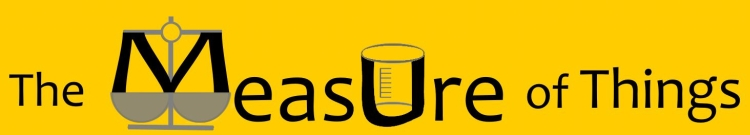Bluebulb Projects presents:Enter a measurement to see comparisons

Equivalents in other units

How big is 0.0000000280 square perches?

Sort Order:
Closest first | Highest first | Lowest first

 It's about as big as a Ballpoint Pen Tip (a.k.a. Biro, a.k.a. ball pen, a.k.a. dot pen) (for medium tip) The most common size of ballpoint pen â€” a medium-tip â€” measures 0.0000000280 square perches. A typical ballpoint pen can write more than 8,500 m (28,000 ft) before running out of ink. It's about one-one-hundredth as big as a Nailhead In other words, 0.0000000280 square perches is 0.00911 times the size of a Nailhead, and the size of a Nailhead is 109.80 times that amount. (for 11-gauge wire nai) An 11-gauge nailhead measures 0.0000030740 square perches. Nails have been used since ancient times; archaeologists have discovered Ancient Egyptian nails that are over 5,000 years old. It's about one-three-hundred-fiftieth as big as a Dime In other words, 0.0000000280 square perches is 0.002811 times the size of a Dime, and the size of a Dime is 355.70 times that amount. (United States ten-cent coin) (a.k.a. Roosevelt dime)The dime, in its current design since 1946, has a diameter of 17.91 mm (0.705 in) and an area of 0.0000099590 square perches. It's about one-four-hundredth as big as a Penny In other words, 0.0000000280 square perches is 0.00248 times the size of a Penny, and the size of a Penny is 403 times that amount. (United States one-cent coin) (a.k.a. Lincoln penny, a.k.a. Cent, a.k.a. One cent piece)The Lincoln penny, in its current design since 1909, has a diameter of 19.05 mm (0.75 in) and an area of 0.00001130 square perches. It's about one-five-hundredth as big as a Nickel In other words, 0.0000000280 square perches is 0.002005 times the size of a Nickel, and the size of a Nickel is 498.80 times that amount. (United States five-cent coin) (a.k.a. Jefferson nickel)The nickel, in its current design since 1938, has a diameter of 21.21 mm (0.835 in) and an area of 0.000013970 square perches. It's about one-five-hundredth as big as a Postage Stamp In other words, the size of a Postage Stamp is 500 times 0.0000000280 square perches. (average; United States dimensions)A typical postage stamp measures an average of 0.0000200 square perches. One of the most prized stamps among collectors is the US-issued "Inverted Jenny" stamp printed in 1918, which features an upside-down image of Curtiss JN-4 ("Jenny") airplane; a single stamp sold for \$977,500 at a 2007 auction. It's about one-six-hundred-fiftieth as big as a Quarter In other words, 0.0000000280 square perches is 0.001532 times the size of a Quarter, and the size of a Quarter is 652.70 times that amount. (United States quarter-dollar coin) (a.k.a. Quarter dollar, a.k.a. Washington quarter, a.k.a. two bits)The George Washington quarter, in circulation in various forms since 1932, has a diameter of 24.26 mm (0.955 in) and an area of 0.000018270 square perches.